# Lenses and Optics

## Purely functional references for traversals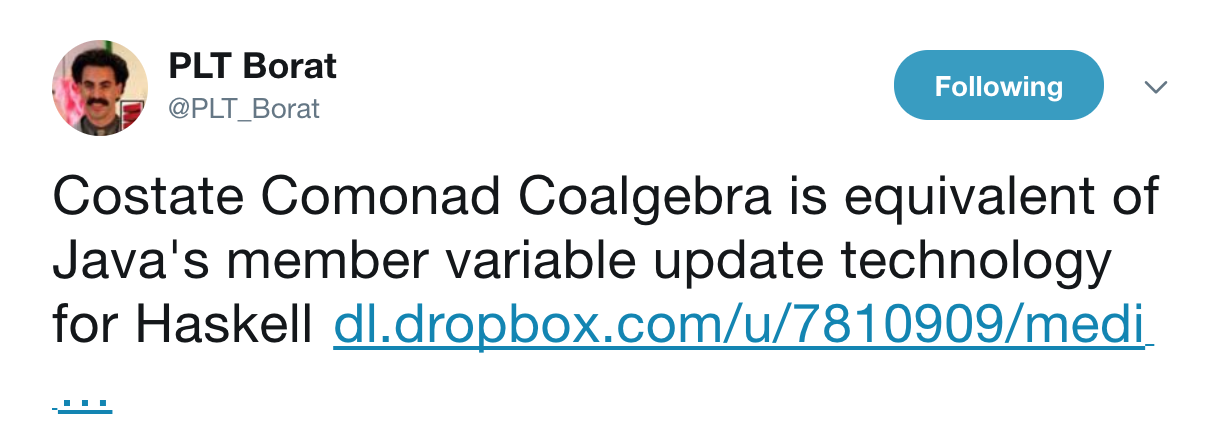# Motivation

## Promoting immutable programming.

Immutability simplifies a lot of concurrency woes.

However it makes working with certain data structures very difficult. Eg: Maps

Maps are ubiquitous.

• JSON
• OOP classes
• Caches
• Databases
• ..

Nearly everything useful you interact with has a map like API

Lets look at some problems working with Haskell records.

A very simple use case

``````
data Person = P { name   :: String
, salary :: Int
}

, city :: String
, postcode :: String}``````

Let us try to change the name of the person.

``````setName :: String -> Person -> Person
setName n P { name = n'
, salary = s}
= P { name = n
, salary = s}``````

Lets try to change his address

``````setPostcode :: String -> Person -> Person
setPostcode pc P { name = n
, city = c
, postcode = p}
, salary = s}
= P { name = n
, city = c
, postcode = pc}
, salary = s}``````

This is starting to look ugly

A real world example from a medical site:

``````{
"problems": [{
"Diabetes":[{
"medications":[{
"medicationsClasses":[{
"className":[{
"associatedDrug":[{
"name":"asprin",
"dose":"",
"strength":"500 mg"
}],
"associatedDrug#2":[{
"name":"somethingElse",
"dose":"",
"strength":"500 mg"
}]
}],
"className2":[{
"associatedDrug":[{
"name":"asprin",
"dose":"",
"strength":"500 mg"
}],
"associatedDrug#2":[{
"name":"somethingElse",
"dose":"",
"strength":"500 mg"
}]
}]
}]
}],
"labs":[{
"missing_field": "missing_value"
}]
}],
"Asthma":[{}]
}]}``````

To change the `strength` of a medicine, which is buried 6 level deep, we have to reconstruct the entire record.

This will cost you the entire reconstruction `time` and `space`!!

Don't even bother trying!!

# Introducing Lens

Hassle free data traversals à la Edward Kmett

## The Hack

(.) in Java acts as an accessor

``employee.setAddress.setPostcode("NG7 2AW")``

(.) in Haskell acts as function composition

``(.) :: (b -> c) -> (a -> b) -> a -> c``

Lens composes functions in a way, that presents a seemingly MUTABLE API, for immutable programming, via the magic of function composition

## Let's try to build a basic Lens type

A Lens primary comprises of 3 functions

• view
• set
• modify

`view`

Given a structure we want to `view` or "focus" on a particular part of the structure

`set`

Given a particular value to be set and a structure we want to create a new structure with that value `set`

`modify`

Given a function to `modify` a part of the structure we want to apply that function to the part of the structure and return the structure

## Lets write the types!

``````data LensR s a
= L { view   :: s -> a
, set    :: a -> s -> s
, modify :: (a -> a) -> s -> s
}``````

``````data LensR s a
= L { view     :: s -> a
, set      :: a -> s -> s
, modify   :: (a -> a) -> s -> s
, modifyIO :: (a -> IO a) -> (s -> IO s)
}``````

What about interactions which can fail?

``````data LensR s a
= L { view     :: s -> a
, set      :: a -> s -> s
, modify   :: (a -> a) -> s -> s
, modifyIO :: (a -> IO a) -> (s -> IO s)
, modifyM  :: (a -> Maybe a) -> (s -> Maybe s)
}``````

``````data LensR s a
= L { view     :: s -> a
, set      :: a -> s -> s
, modify   :: (a -> a) -> s -> s
, modifyIO :: (a -> IO a) -> (s -> IO s)
, modifyM  :: (a -> Maybe a) -> (s -> Maybe s)
, modifyS  :: (a -> State s a) -> (s -> State s s)
}``````

Can you notice a pattern?

Every interaction is falling into this pattern

``modifyFoo :: (a -> Foo a) -> (s -> Foo s)``

And if you really squint your eyes:

``set :: a -> s -> s``

`view` is the only function whose types do not visibly seem similar

Let us try to capture the entire pattern using one function excluding `view`

``type Lens f s a = (a -> f a) -> (s -> f s)``

A short digression

## Jargon time

• Higher Rank Polymorphism
• Functors

Constraints liberate. Liberties constrain.

Eg:

``````foo :: Num a => a -> a    foo :: Bool a => a -> a
foo 5 = 6                 foo True  = False
foo 6 = 8                 foo False = True
foo ...                   foo ...``````

Now lets liberate the type level

``````foo :: forall a . a -> a
foo x = x``````

## Functors

A functor is a Higher Kinded Type

``````class Functor f where
fmap :: (a -> b) -> f a -> f b``````

Higher kinded types take one or more types and return a type.

`IO`, `Maybe`, `State` are all monads.

Functors are weaker than monads but they have laws

``````fmap id = id
fmap (f . g) = fmap f . fmap g``````

## Back to lens

Lets combine higher rank polymorphism and functors

# Van Laarhoven Lens

One function to rule them all

``type Lens s a = forall f . Functor f => (a -> f a) -> (s -> f s)``

Claim: `view`, `set` and `modify` are all captured by this function.

Lets look at `view`

``view :: s -> a``
``type Lens s a = forall f . Functor f => (a -> f a) -> (s -> f s)``

Seriously? How on earth?

Say hello to the `Const` type

``````newtype Const v a = Const v

getConst :: Const v a -> v
getConst (Const x) = x

instance Functor (Const v) where
fmap f (Const x) = Const x``````
``````view :: forall s a . Lens s a -> s -> a
view ln s = ?``````
``````λ> :t Const
Const :: v -> Const v a``````

Remember:

``type Lens s a = forall f . Functor f => (a -> f a) -> (s -> f s)``

Now,

``````(a -> f a) ≡ Const ≡ (a -> Const a a)
s ≡ s``````

So

``ln Const s ≡ Const a s``

Hence

``````view :: forall s a . Lens s a -> s -> a
view ln s = getConst  \$ ln Const s``````

Similarly,

Using the identity functor we can get

``````set :: forall s a . Lens s a -> a -> s -> s
set ln x s = runIdentity \$ ln (\_ -> Identity x) s``````
``````modify :: forall s a . Lens s a -> (a -> a) -> s -> s
modify ln f s = runIdentity \$ ln (Identity . f) s``````

## LENSES COMPOSE!!

Because it is just a function

Remember the old medical site example

Lets compose our way into its depth:

``````Lens Problem Disease .
Lens Disease Medication .
Lens Medication MedicationClass .
Lens MedicationClass Classname .
Lens Classname Drug .
Lens Drug String
= Lens Problem String``````

That was just plain old function composition!

And making a Lens can be incredibly automated

``````import Control.Lens.TH

\$(makeLenses ''Problem)
\$(makeLenses ''Disease)
\$(makeLenses ''Medication)
...``````

And if you don't like metaprogramming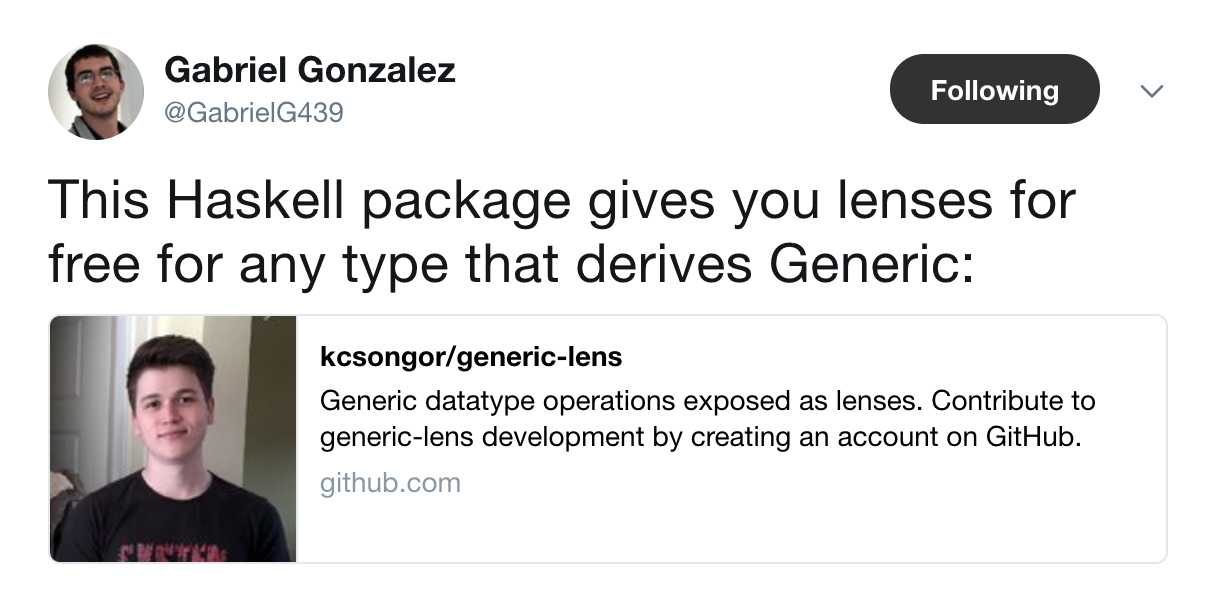Generalizing the Lens type

``type Lens s t a b = forall f . Functor f => (a -> f b) -> s -> f t``

Is that all?

How big is the functor family?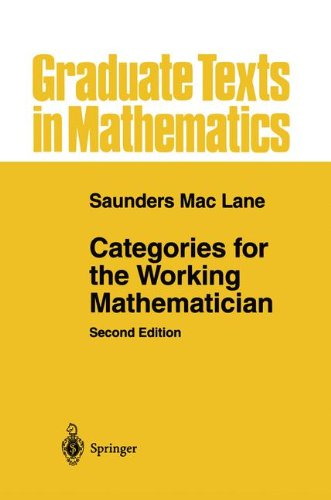The functor hierarchy existing in Haskell excluding lots of interesting categories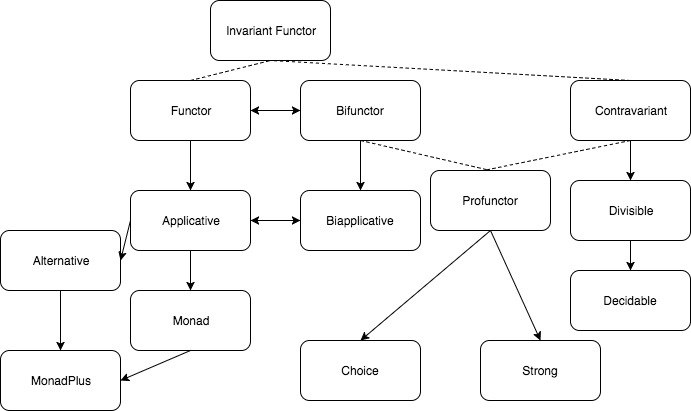Constraining this in various ways

``type Lens s t a b = forall f . (??) => (a -> f b) -> s -> f t``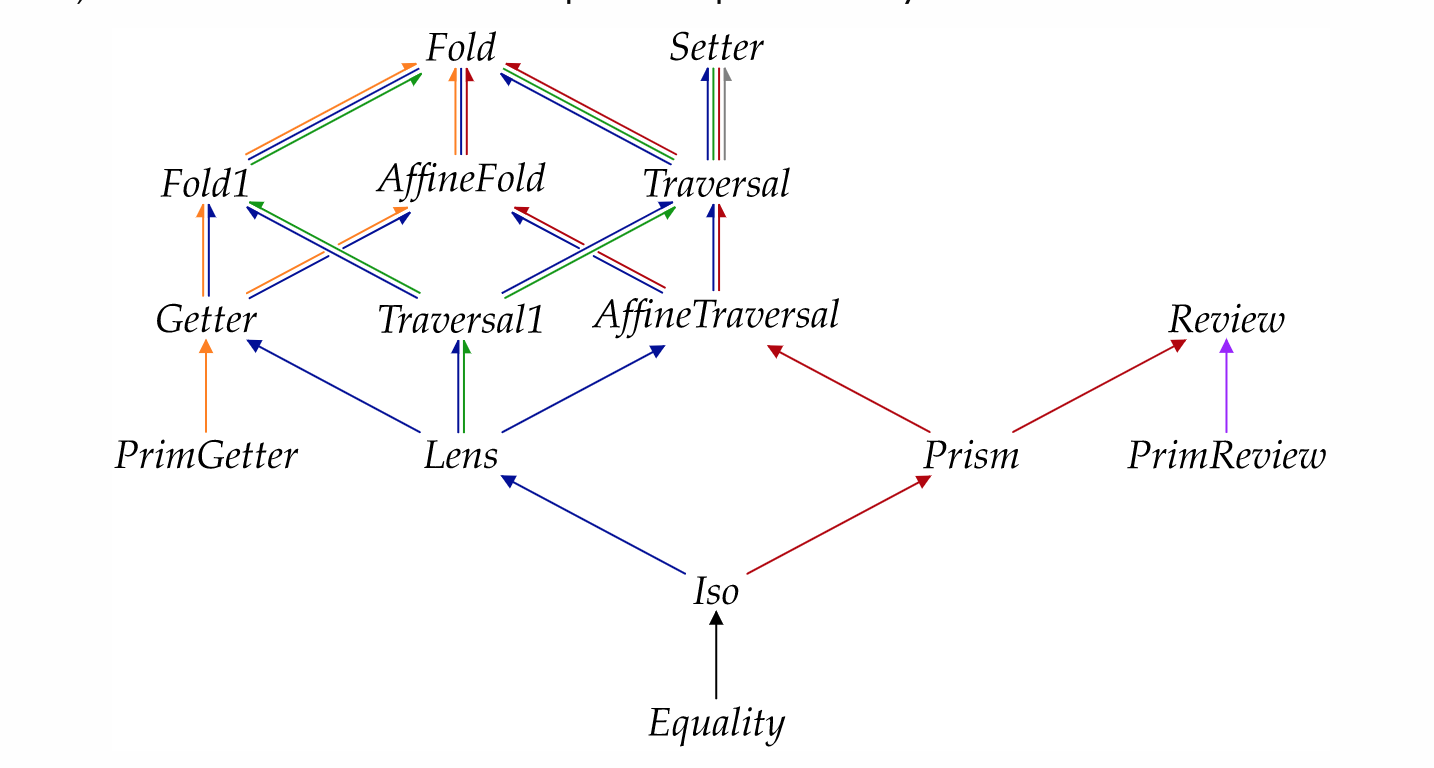But thats too much jargon for my taste!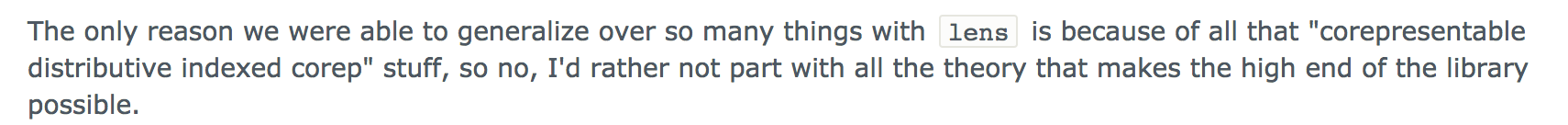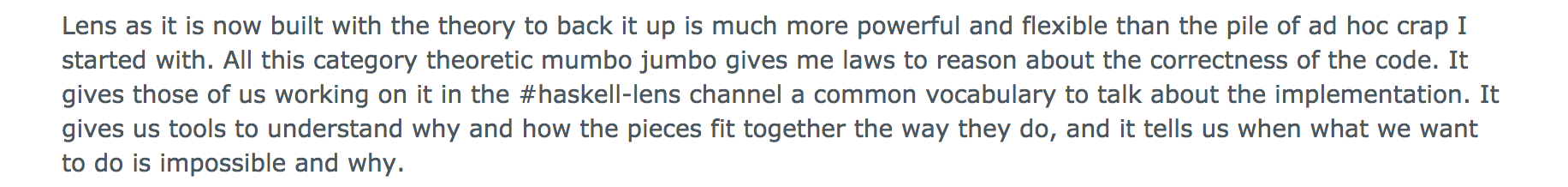## Lens inlines aggresively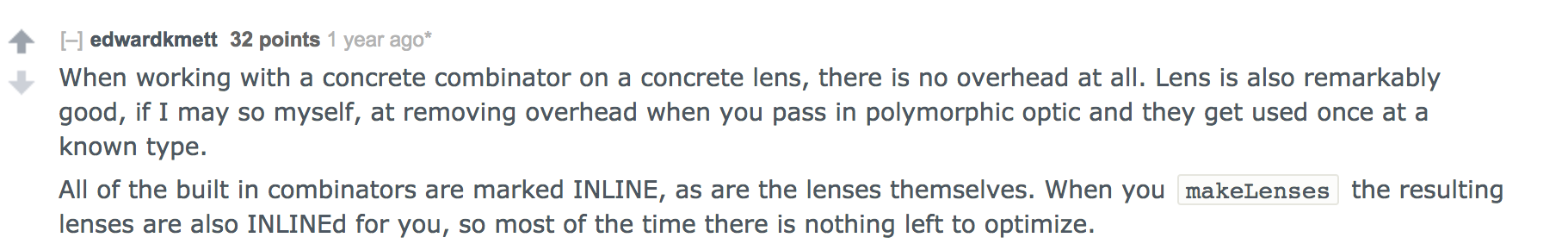Inlining example from SPJ's talk

``````view :: Lens s a -> (s -> a)
view ln = getConst . ln Const

name :: Lens Problem String
name elt_fn (P n s)
= fmap (\n' -> P n' s) (elt_fn n)``````
``````view name (P {_name = "Fred", _salary = 100})
-- inline view
= getConst (name Const (P {_name = "Fred"}))
-- inline name
= getConst (fmap (\n' -> P n' 100) (Const "Fred"))
-- fmap f (Const x) = Const x
= getConst (Const "Fred")
-- getConst (Const x) = x
= "Fred"``````

`view`, `set` and `modify` inlines almost everything

Giving `O(1)` time complexity

Same as Java, C++ accessors

But the actual win is in space complexity!

A normal map implementation would have linear space growth

Inlining leads to `O(1)` space complexity for updates in persistent data structures

``type Traversal s t a b = forall f . Applicative f => (a -> f b) -> (s -> f t)``

Applicative = parallel

Time Complexity `O(1)` but faster on multiple cores

### This is the tip of the iceberg:

• Profunctor Optics

• Indexed Optics

• ...

### Example of how crazy it can get: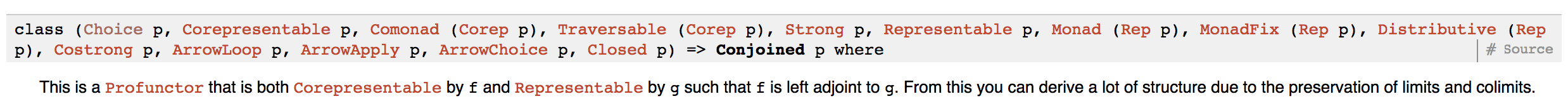### Entire code up on github

https://github.com/Abhiroop/opto

Future work will be based on this: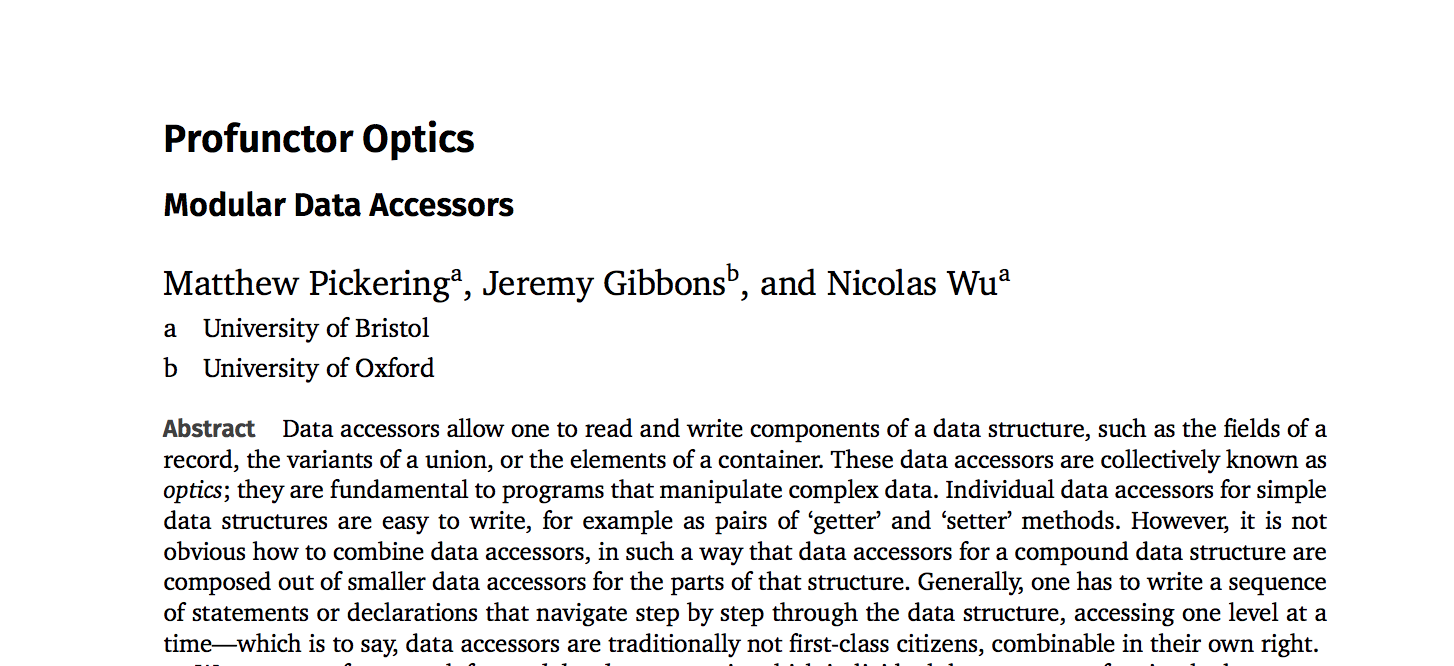References

Special Thanks to

Prof. Jeremy Gibbons,

for clarifying my doubts over the mail on a Sunday

Others:

Edward Kmett, NYC Haskell Meetup Talk

Simon Peyton Jones, Haskell Exchange 2013

Blog post series by Jakub Arnold https://blog.jakuba.net